#### 期刊菜单

Realization of 3D-Based Face Synthesis and Replacement System
DOI: 10.12677/SEA.2022.113056, PDF, HTML, XML, 下载: 72  浏览: 677

Abstract: Face synthesis, also known as face replacement, while maintaining the identity of the person, aims to seamlessly and realistically fuse the face in the source image to the target image. This paper proposes an automated face synthesis and replacement system, which uses labeled images to synthesize new images that users are interested in, and realizes applications such as virtual hair test and virtual fitting. The input of the system is a single face image and query text, where the query text is used to search for relevant images and construct a set of target images to be replaced. In order to improve the effect of face synthesis, this paper firstly reconstructs a 3D face model through face images, completes the face pose and expression migration in 3D space, and then estimates the ambient lighting through face image decomposition to realize the re-illumination of face images, and finally realize the image fusion of the face through the image fusion technology. Experiments show that our method can synthesize more realistic face images.

1. 引言

2. 系统流程介绍Figure 1. Automatic face replacement pipeline

3. 系统模块介绍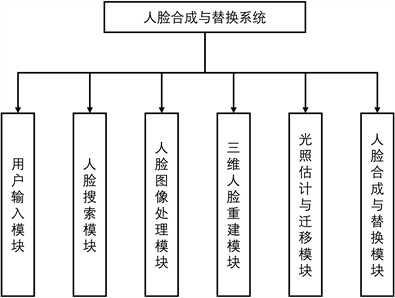Figure 2. System module

1) 用户输入模块：用户输入模块用来向用户提供输入接口，接收用户输入，包括文本输入和图片路径选择。

2) 人脸搜索模块：该模块负责从本地库或互联网中查找与输入文本关键字相关的人脸图像。系统运行后，人脸搜索模块从前端用户界面获取文本进行判断。若文本不为空，则从本地库或缓存图片库中查找与该文本具有相符标签的图像集合。若不存在这类的图像，则通过搜索引擎接口从互联网中查询相关图像，缓存到本地库后返回该图像集合。具体流程如图3所示。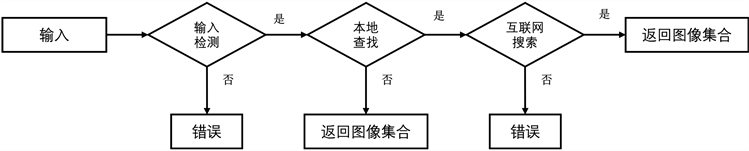Figure 3. Face search processFigure 4. Image preprocessing process

4) 人脸重建模块：人脸重建模块用于生成与人脸图像相对应的三维人脸模型。如图5所示，人脸重建模块包含了三个子模块：三维人脸参数回归模块、三维人脸模型生成模块和渲染模块。在三维人脸参数回归模块中，分别获得源图像和待替换的目标人脸图像集合对应的三维形变模型(3D Morphable Model, 3DMM)  系数，通过将源图像的三维形变模型表情系数和姿态系数修改为目标图像集合人脸的表情系数和姿态系数，并保持形状系数不变，可实现三维人脸的表情与姿态迁移。三维人脸模型生成模块用来根据形变模型系数生成三维人脸模型。渲染模块根据姿态系数定义相机位置，将三维人脸模型渲染为二维图像。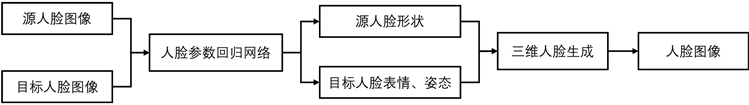Figure 5. Face reconstruction process

5) 光照处理模块：基于三维形变模型方法可以重建人脸的形状和表情，但对于人脸的纹理重建效果不好，只能生成较为光滑的人脸纹理，无法恢复胡须、皱纹等高频细节。因此，文本将原始图像中的人脸颜色作为纹理映射到三维人脸模型，但原始图像的人脸颜色包含了光照等信息，并不是人脸真正的颜色。不同的图像所处的环境光照不同，也会呈现出不同的人脸光影效果。光照处理模块用于完成人脸图像的光照估计与迁移任务，生成在目标图像光照下的人脸图像，流程如图6所示。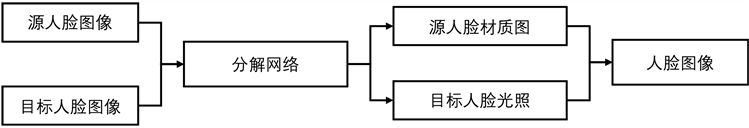Figure 6. Relighting process

6) 人脸替换模块：人脸替换模块用来实现最终的人脸融合与替换，将重光照后的源图像映射到三维模型中，并将渲染后的人脸融合到目标图像的待替换区域。

4. 系统模块实现

4.1. 人脸图像处理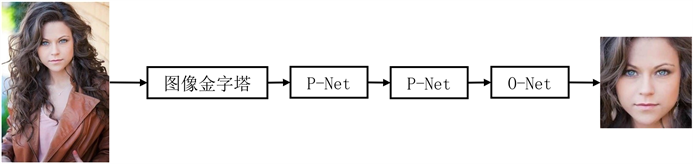Figure 7. MTCNN algorithm processTable 1. Key point corresponding position

$\left[\begin{array}{c}u\\ v\\ 1\end{array}\right]=\left[\begin{array}{ccc}\frac{1}{dx}& 0& {u}_{0}\\ 0& \frac{1}{dy}& {v}_{0}\\ 0& 0& 1\end{array}\right]\left[\begin{array}{cccc}\frac{f}{{z}_{c}}& 0& 0& 0\\ 0& \frac{f}{{z}_{c}}& 0& 0\\ 0& 0& \frac{1}{{z}_{c}}& 0\end{array}\right]\left[\begin{array}{cc}R& t\\ {0}^{\text{T}}& 1\end{array}\right]\left[\begin{array}{c}{x}_{w}\\ {y}_{w}\\ {z}_{w}\\ 1\end{array}\right]$ (1)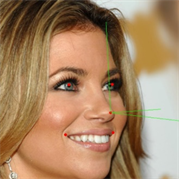Figure 8. Pose estimation result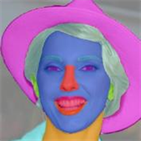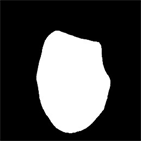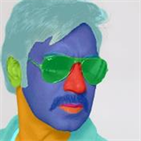Figure 9. Face segmentation result

4.2. 人脸重建与密集对齐

$S=\stackrel{¯}{S}+{A}_{id}{\alpha }_{id}+{A}_{exp}{\alpha }_{exp}$ (2)

${V}_{2d}\left(p\right)=f\ast Pr\ast R\ast \left(\stackrel{¯}{S}+{A}_{shp}{\alpha }_{shp}+{A}_{exp}{\alpha }_{exp}\right)+{t}_{2d}$ (3)

${V}_{2d}\left(p\right)$ 表示人脸重建和投影函数，将三维人脸顶点投影到二维平面，获得其在二维平面的位置坐标，f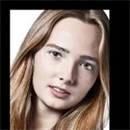输入图像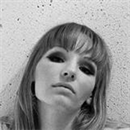目标图像a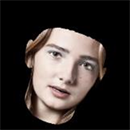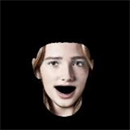目标图像b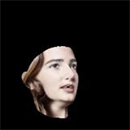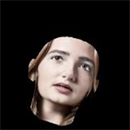目标图像c

Figure 10. Face reconstruction result

4.3. 光照估计与迁移

$E\left(n\right)=\underset{l=0}{\overset{\infty }{\sum }}\underset{m=-l}{\overset{l}{\sum }}{A}_{l}{L}_{l}^{m}{Y}_{l}^{m}\left(n\right)\approx \underset{l=0}{\overset{2}{\sum }}\underset{m=-l}{\overset{l}{\sum }}{A}_{l}{L}_{l}^{m}{Y}_{l}^{m}\left(n\right)$ (4)

$I\left(x,y\right)=\rho \left(x,y\right)E\left(n\right)$ (5)

$I\left(x,y\right)\approx \underset{l=0}{\overset{2}{\sum }}\underset{m=-l}{\overset{l}{\sum }}\rho \left(x,y\right){A}_{l}{L}_{l}^{m}{Y}_{l}^{m}\left(\alpha \left(x,y\right),\beta \left(x,y\right)\right)$ (6)输入图像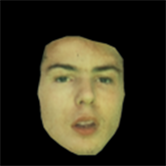源光照下的人脸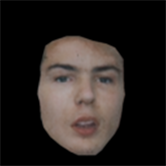重光照后的人脸

Figure 11. Relighting result

5. 系统界面实现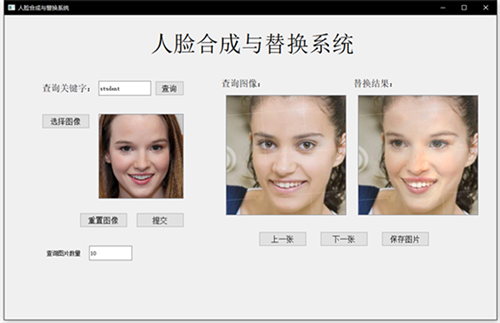Figure 12. System interface

6. 结果展示SourceTarget 1Target 2Target 3Target 4Result 1Result 2Result 3Result 4SourceTarget 1Target 2Target 3Target 4Result 1Result 2Result 3Result 4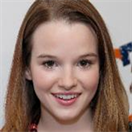SourceTarget 1Target 2Target 3Target 4Result 1Result 2Result 3Result 4

Figure 13. Face synthesis and replacement result

  Blanz, V. and Vetter, T. (1999) A Morphable Model for the Synthesis of 3d Faces. Proceedings of the 26th Annual Conference on Computer Graphics and Interactive Techniques, Los Angeles, 8-13 August 1999, 187-194. https://doi.org/10.1145/311535.311556  Zhang, K., Zhang, Z., Li, Z., et al. (2016) Joint Face Detection and Alignment Using Multitask Cascaded Convolu-Tional Networks. IEEE Signal Processing Letters, 23, 1499-1503. https://doi.org/10.1109/LSP.2016.2603342  Kaehler, A. and Bradski, G. (2016) Learning OpenCV 3: Computer Vision in C++ with the OpenCV library. O’Reilly Media, Inc., Newton.  Lee, C.H., Liu, Z., Wu, L., et al. (2020) Maskgan: Towards Diverse and Interactive Facial Image Manipulation. Proceedings of the IEEE/CVF Conference on Computer Vision and Pattern Recognition, Seattle, 13-19 June 2020, 5549-5558. https://doi.org/10.1109/CVPR42600.2020.00559  Yu, C., Wang, J., Peng, C., et al. (2018) Bisenet: Bilateral Segmentation Network for Real-Time Semantic Segmentation. In: Ferrari, V., Hebert, M., Sminchisescu, C. and Weiss, Y., (Eds.), Computer Vision-ECCV 2018. ECCV 2018. Lecture Notes in Computer Science, Heidelberg, Cham, 325-341. https://doi.org/10.1007/978-3-030-01261-8_20  Fan, M., Lai, S., Huang, J., et al. (2021) Rethinking Bisenet for Real-Time Semantic Segmentation. Proceedings of the IEEE/CVF Conference on Computer Vision and Pattern Recognition, Nashville, 20-25 June 2021, 9716-9725. https://doi.org/10.1109/CVPR46437.2021.00959  Zhu, X., Liu, X., Lei, Z., et al. (2017) Face Alignment in Full Pose Range: A 3d Total Solution. IEEE Transactions on Pattern Analysis and Machine Intelligence, 41, 78-92. https://doi.org/10.1109/CVPR46437.2021.00959  Frolova, D., Simakov, D. and Basri, R. (2004) Accuracy of Spherical Harmonic Approximations for Images of Lambertian Objects under Far and Near Lighting. In: Pajdla, T. and Matas, J., (Eds.), Computer Vision-ECCV 2004. ECCV 2004. Lecture Notes in Computer Science, Springer, Heidelberg, 574-587. https://doi.org/10.1007/978-3-540-24670-1_44  Sengupta, S., Kanazawa, A., Castillo, C.D., et al. (2018) Sfsnet: Learning Shape, Reflectance and Illuminance of Facesin the Wild. Proceedings of the IEEE Conference on Computer Vision and Pattern Recognition, Salt Lake City, 18-23 June 2018, 6296-6305. https://doi.org/10.1109/CVPR.2018.00659  Willman, J.M. (2022) Creating Custom Widgets. Beginning PyQt. Apress, Berkeley, 341-358. https://doi.org/10.1007/978-1-4842-7999-1_12